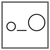Fuddlepixel coz tech is fun();

# Blog

##### Miscellaneous Operators and Operator Precedence

Let us discuss the some important miscellaneous operators available in C language to carry some important stuffs and also the operator precedence.

##### Bitwise Operators

Let us introduce ourselves to the Bitwise Operators, which are used for manipulating bits by shifting and performing binary operations.

##### Mathematical Reasoning

Let us discuss how maths can perform logical operations and not only calculations. It is the subject called mathematical reasoning to do logic in maths !

##### Binary Number System

Let us discuss the Binary Number System, the zero and one coding system which is the actual language of computer and electronics !

##### Logical Operators

Let us discuss the logical operators through which we can perform logical operations in the C programming language, that makes coding fun !

##### Inside Logic Gates - The Electronic Logic

Let us discuss how electronic equipment really work with logic. How Logic Gates works. The electronic logic forms the real brain of computer systems.

Top